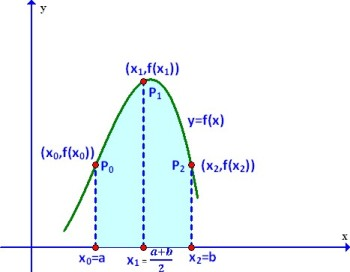# Simpson’s 1/3 Rule – C Program

Simpson’s Rule is a Numerical technique to find the definite integral of a function within a given interval.

The function is divided into many sub-intervals and each interval is approximated by a quadratic curve. And the area is then calculated to find the integral. The more is the number of sub-intervals used, the better is the approximation.
NOTE: The no. of sub-intervals should be EVEN.### Formula Used:$\int_a^bf(x)dx \approx \frac{h}{3}(f(a)+4f(a+h)+2f(a+2h)+4f(a+3h)+2f(a+4h)+....+f(b))$$\implies \int_a^bf(x)dx \approx \frac{h}{3}(f(a)+4f(x_1)+2f(x_2)+4f(x_3)+2f(x_4)+...+f(b))$

where$x_i=a+ih$ for$i=1,2,....n-1$ and$h=(b-a)/n$
The following C Program uses the Simpson’s 1/3 Rule to find the definite integral of a function.
Users, will have to change the function f in the following program to the function whose integral they want to find.

### PROGRAM (Simple Version):

/*********************************
*******SIMPSON'S 1/3 RULE********
2017 (c) Manas Sharma - https://bragitoff.com
********************************/
#include<stdio.h>
#include<math.h>

/* Define the function to be integrated here: */
double f(double x){
return x*x;
}

/*Program begins*/
main(){
int n,i;
double a,b,h,x,sum=0,integral;
/*Ask the user for necessary input */
printf("\nEnter the no. of sub-intervals(EVEN): ");
scanf("%d",&n);
printf("\nEnter the initial limit: ");
scanf("%lf",&a);
printf("\nEnter the final limit: ");
scanf("%lf",&b);
/*Begin Simpson's Procedure: */
h=fabs(b-a)/n;
for(i=1;i<n;i++){
x=a+i*h;
if(i%2==0){
sum=sum+2*f(x);
}
else{
sum=sum+4*f(x);
}
}
integral=(h/3)*(f(a)+f(b)+sum);
/*Print the answer */
printf("\nThe integral is: %lf\n",integral);
}



### OUTPUT:

The above program returns a better approximation to the interval as the number of sub-intervals is increased. This might work for some applications, however, sometimes one might not want to deal with the number of sub-intervals, but rather the accuracy upto a certain decimal places. What I mean by accuracy is that sometimes you might just want the approximate value of integral upto a few decimal place. So you will have to keep on increasing the number of sub-intervals and check the value of the integral. If the integral for two subsequent no. of sub-intervals is within the accuracy/tolerance limit given by the user(or set by you) then the integral should be printed out.

The following program illustrates the process of achieving what I just explained and also uses a function called ‘simpsons’ that handles the integration part.

### PROGRAM (Better Version):

/************************************************
*******SIMPSON'S 1/3 RULE USING FUNCTION********
2017 (c) Manas Sharma - https://bragitoff.com
***********************************************/
#include<stdio.h>
#include<math.h>

/* Define the function to be integrated here: */
double f(double x){
return x*x;
}

/*Function definition to perform integration by Simpson's 1/3rd Rule */
double simpsons(double f(double x),double a,double b,int n){
double h,integral,x,sum=0;
int i;
h=fabs(b-a)/n;
for(i=1;i<n;i++){
x=a+i*h;
if(i%2==0){
sum=sum+2*f(x);
}
else{
sum=sum+4*f(x);
}
}
integral=(h/3)*(f(a)+f(b)+sum);
return integral;
}

/*Program begins*/
main(){
int n,i=2;
double a,b,h,x,sum=0,integral,eps,integral_new;

/*Ask the user for necessary input */
printf("\nEnter the initial limit: ");
scanf("%lf",&a);
printf("\nEnter the final limit: ");
scanf("%lf",&b);
printf("\nEnter the desired accuracy: ");
scanf("%lf",&eps);
integral_new=simpsons(f,a,b,i);

/* Perform integration by simpson's 1/3rd for different number of sub-intervals until they converge to the given accuracy:*/
do{
integral=integral_new;
i=i+2;
integral_new=simpsons(f,a,b,i);
}while(fabs(integral_new-integral)>=eps);

/*Print the answer */
printf("\nThe integral is: %lf for %d sub-intervals.\n",integral_new,i);
}


### OUTPUT:[wpedon id="7041" align="center"]

## 8 thoughts on “Simpson’s 1/3 Rule – C Program”

The code is not working properly ITIS showing nan error

1. Hi Suman,
Did you run the program as it is, or did you make any changes? If you made changes them what were those?

2. Sir please solve the problem …integration 0 to 1 solve sinx by simpson’s 3/8 rule…please sir

3. Good day sir, can you explain how “n” was used in the “better version” of the program? Thank you!

1. Actually it was silly to call it ‘better’. The difference is that in this version I am asking the user about how accurate the integral must be. And I keep calculating the integral with increasing values of n until the change in the integral is within the desired accuracy.

4. Find ∫0 𝜋 cos 𝑥 𝑑𝑥 0 by Simpson’s 3/8 rule using C programming.
plz solve

5. Solve the following system of equation by Gaussian Elimination method.
2𝑥 − 6𝑦 + 8𝑧 = 24
5𝑥 + 4𝑦 − 3𝑧 = 2
3𝑥 + 𝑦 + 2𝑧 = 16

plz solve the question and gmail me